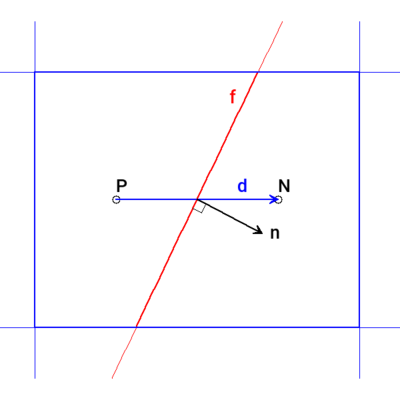# Code of the Week #6: laplacian(rAU, p) linear corrected

laplacianSchemes
{
laplacian(rAU, p) Gauss linear corrected;
}


laplacianSchemes 是在 system/fvSchemes 文件里的子字典，指定 laplacian 项的离散格式，其语法是

laplacianSchemes
{
default         none;
}


\nabla\cdot(\Gamma\nabla \phi) = \Gamma\nabla^2 \phi = \Gamma\left(\frac{\partial^2}{\partial x_1^2} \phi + \frac{\partial^2}{\partial x_2^2} \phi + \frac{\partial^2}{\partial x_3^2} \phi\right)

FVM 方法

\nabla\cdot \left( \Gamma \nabla \phi \right) = \frac{1}{V}\int_V \nabla\cdot \left( \Gamma \nabla \phi \right)dV = \frac{1}{V} \oint_S \Gamma \nabla \phi d\vec{S} = \frac{1}{V} \sum_i^{\mathrm{nFace}}\left(\nabla{\phi}\right)_{f,i} \cdot \Gamma_f \vec{S}_{f,i}

Laplacian 項离散为该控制体所有面的面法向量与該面上 \phi 的梯度的点积之和。从体心到面心需要一种插值方法 (interpolationScheme) 。

\nabla_f^\perp \phi = \vec{n} \cdot \left( \nabla \phi \right)_fd 和 n 向量间的夹角代表了非正交性。

\nabla_f^\perp \phi = \underbrace{\alpha \frac{\phi_P - \phi_N}{| \vec{d} |}}_{\mathrm{implicit}} + \underbrace{\left( \hat{\vec{n}} - \alpha \hat{\vec{d}} \right) \cdot \left( \nabla \phi \right)_f}_{\mathrm{explicit\ correction}}

\psi = \begin{cases} \begin{array}{ll}% 0 & \text{不修正，相当于 uncorrected}\\ 0.333 & \text{非正交修正} \leqslant 0.5\times 正交量\\ 0.5 & \text{非正交修正} \leqslant 正交量\\ 1 & \text{非正交修正,相当于 corrected} \end{array} \end{cases}

laplacianSchemes
{
//default         Gauss linear uncorrected;
laplacian(gamma,phi) Gauss linear limited corrected 0.33;
}


laplacianSchemes
{
//default         Gauss linear uncorrected;
laplacian(gamma,phi) Gauss linear limited 0.33;
}


2 Likes

1） 非正交角度是否为 \theta ? \alpha=\frac{1}{cos(\theta)} 是系数 ？
2）limited 系数 \psi 直接乘在显示修正项上 ？即：

\nabla_{f}^{\perp} \phi=\underbrace{\alpha \frac{\phi_{P}-\phi_{N}}{|\vec{d}|}}_{\text {implicit }}+\underbrace{\psi(\hat{\vec{n}}-\alpha \hat{\vec{d}}) \cdot(\nabla \phi)_{f}}_{\text {explicit correction }}

3）一般情况下 \psi=0.33 ，不管角度大小都适用 ？

4） nNonOrthCorr 循环好像和这个修正不是一回事 ？

//网格非正交循环
for (int nonOrth=0; nonOrth<=nNonOrthCorr; nonOrth++)
{
fvScalarMatrix pEqn
(
fvm::laplacian(rUA, p) == fvc::div(phi)  // 压力泊松方程，此处间接使用 Rhie-Chow 插值原理
);

pEqn.setReference(pRefCell, pRefValue);
pEqn.solve();               // 求解压力泊松方程

if (nonOrth == nNonOrthCorr)
{
phi -= pEqn.flux();   // 方程 (7b)，使用求解的压力场修正通量场，即在最后一次修正的时候使通量守恒，